Area

#### Area is the amount of surface of a shape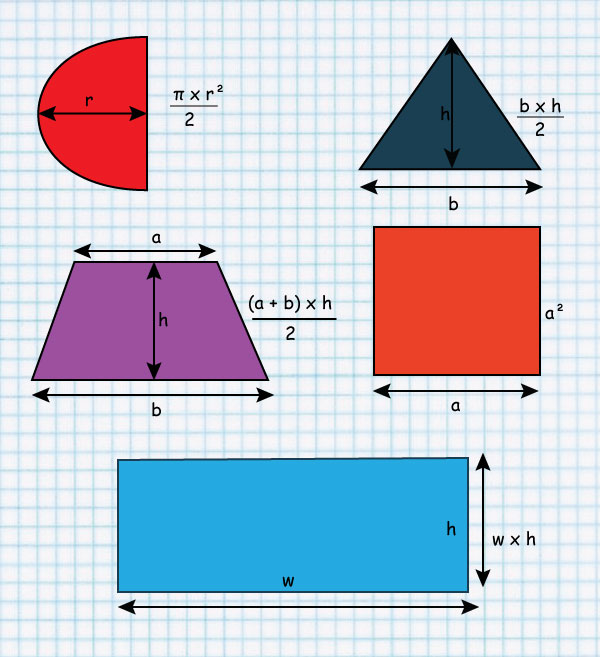When we refer to a solid such as a cube, the amount of surface is called its surface area.

Area is usually measured in the following units:

• Centimetre square (cm²)
• Metre square (m²)
• Kilometer square (km²)

If 1 m = 100 cm then

1m² = 1m x 1 m

therefore 1m² = 100 cm x 100 cm

= 10 000 cm²

The following shows how the units of area are related.

• 10 000 cm² = 1 m²
• 10 000 m² = 1 ha
• 100 m² = 1 are
• 100 are = 1 ha
• 100 ha = 1 km²

We can use the relationships given above to work out the area of specific and combined shapes.

Similarly, we can work out the surface area of solids such as cubes, cuboids, and cylinders.

### Let's learn how to calculate area

Square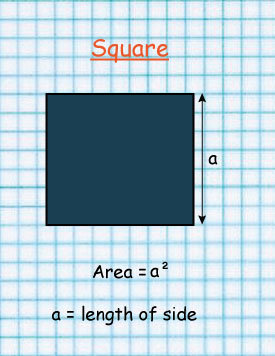#### Rectangle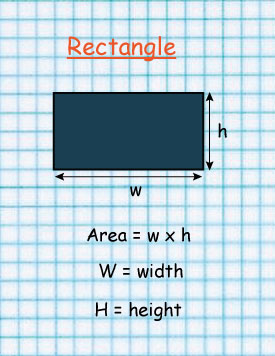#### Triangle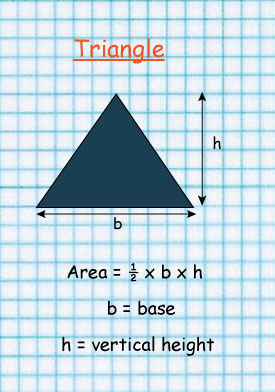Circle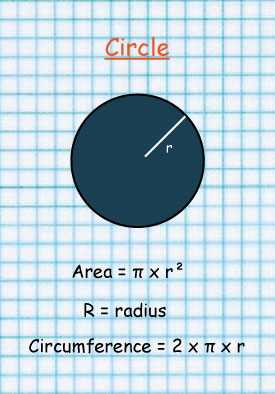Parallelogram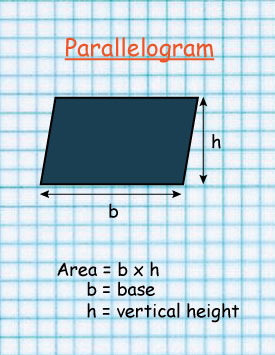#### Trapezium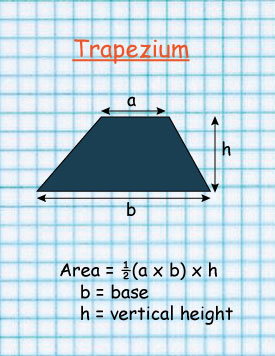#### Can this rectangle become a triangle?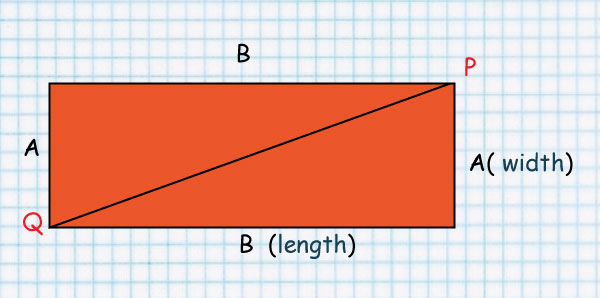Look at line QP.

It splits the rectangle into two equal Triangles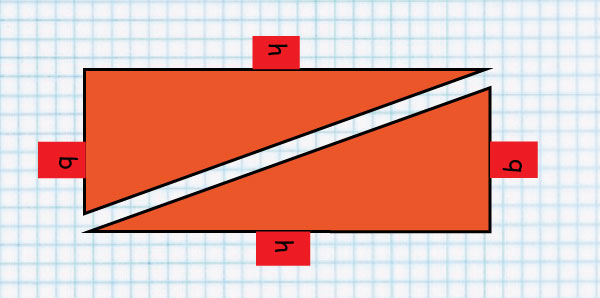That’s why the Area of a Triangle is 1/2 b x h

#### A Circle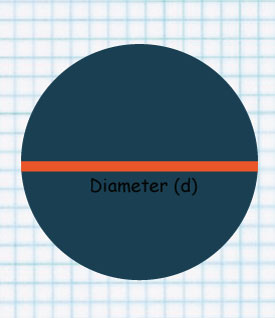Area of a circle is given by:

A = π  ×  r  ×  r

The line at the center is called Diameter.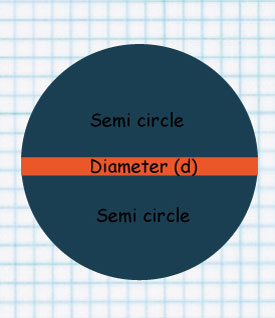Diameter cuts the circle into two equal parts.

Each part is called semi circle.

A semi-circle can be divided into two equal parts to form a Quadrant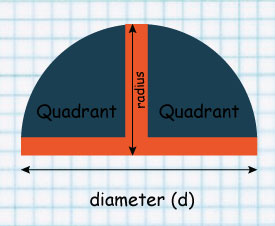When diameter is divided into 2, a Radius is formed.

Below is a song that teaches you more about circles.

#### Example

Calculate the area of the circles below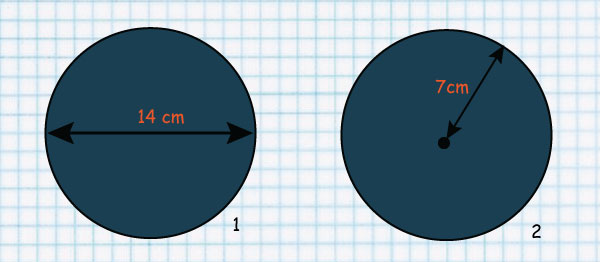#### Solution

Area of a circle 1 =Pie  ×  r  × r

Pie =`22/7`

=  × `14/2` cm ×`14/2` cm

=`22/7` × 7cm × 7cm

=22 × 7cm × 1cm

=154cm2

Area of a circle 2 = Pie ×  r  × r

Pie =`22/7`

= `22/7 ` × `14/2` cm  × `14/2` cm

=`22/7` × 7cm × 7cm

=22 × 7cm × 1cm

=154cm2

The two circle have the same areas.

The first circle has a diameter of 14cm while the second has a radius of 7cm hence a diameter of 14cm

Find the areas of the shaded part of the circle below given that it has a radius of 7cm.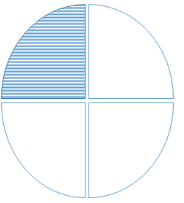Solution

Area of the whole circle

=`22/7` × 7cm × 7cm

=22 × 1cm × 7cm

= 154cm2

However the circle has been divided into 4 equal parts each called a Quadrant

To get areas of the shaded part divide 154cm2 by 4

= `154/4` cm2

=38.5cm2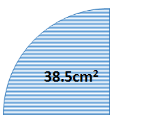#### Parallelogram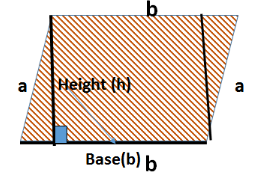The above parallelogram is made up of two right angled triangles and a rectangle.

The two opposite sides are equal

b=b

a=a

The area of a parallelogram is given by:

Base length  ×  height

Find the area of the shaded regions in the figures below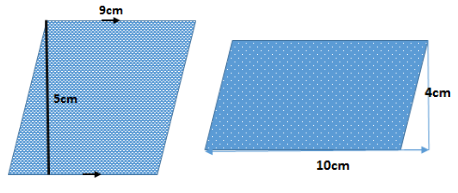Area of a parallelogram = base length X height

a )                                                                             b)

=9cm × 5cm                                                         =10cm × 4cm

= 45 cm squared                                                     = 40 cm squared

#### Trapezium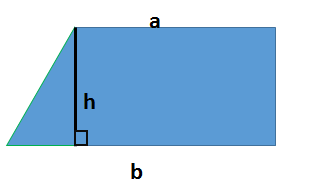The above trapezium is made up of a rectangle and a right angled triangle.

The area of a Trapezium is given by:

½ h  × sum of the two parallel sides(a+b)

Study the image below: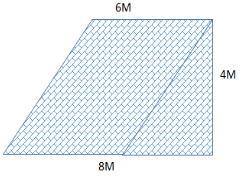What is the area of the shaded region?

Remember

The area of a Trapezium is given by:

½ h × sum of the two parallel sides(a+b)

½ × 4m × (6m + 8m)

2m × (14m)

=28 metres squared.

1. The area of the figure below is 360m2.

Find the  length of a.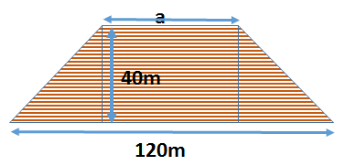2. The figure below represents a plot of land. What is the area of the plot.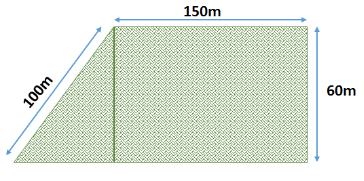1. 60 m

2. 9000 metres squared

The figure below shows a circle of radius 7cm touching the vertices of a square.

What is the area of the shaded part?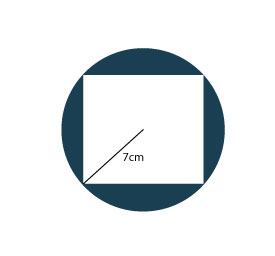Get the area of the circle A = π r^2

= 3.14 × 7 cm × 7 cm

= 153.86 cm squared

Get the area of the square. The radius gives half the length of the side of the square.

To get full length= 7cm × 2

=14cm

Area of a square =S × S

=14cm × 14cm

=196cm squared

Area of the shaded part = Area of the circle - Area of the square

= 196cm squared- 153.86cm squared

= 42.14cm squared

Find the area of the shaded part in the figure below

Take pie = 3.14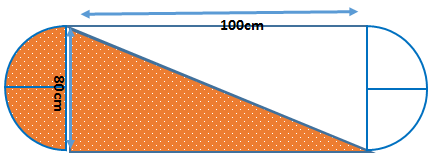The shaded part is made up of a semicircle and a right angled triangle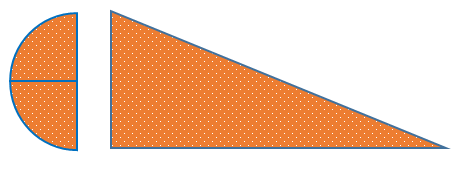Area of the semicircle = (πr^2)/2

80cm = diameter

= 40cm

3.14 x 40cm x 40cm

=5024 cm2/2

=2512cm squared

Area of the triangle = ½ b  x  h

= ½ x 100cm  x  80cm

=50cm  x  80cm

= 4000cm squared

Area of the shaded part = 2512cm squared+ 4000cm squared

= 6512cm squared

The image shows a window of a building in Nairobi.

Calculate the area of the window.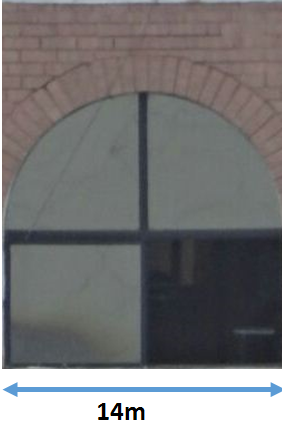#### Solution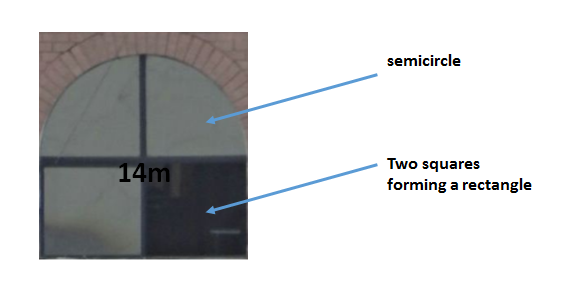Area = area of the rectangle + area of the semicircle

Area of rectangle= l × w

14 m×7 m

=98m2

Area of the semicircle = (πr^2)/2

22/7 ×7 m×7 m

= 154 m2/2

=77 m2

Answer = 98 m2 + 77 m2

=175 m2

## KCPE Topical Questions

Class 7

### Area of varied shapes

1. The perimeter of a rectangular field is 14 m. if one of the sides is 3 m, what is the area of the field?

A. 21 m2       B. 33 m2       C. 42 m2      D. 12 m2

2. A circular cut-out of diameter 14 cm is removed from a square board of length 30 cm. What is the area of the piece of board that remains? (Take 22/7)

A. 856 cm2         B. 154 cm2        C. 746 cm2           D. 284 cm2

3. The figure below shows a square WXYZ drawn inside a circle centre O. The radius of the circle is 3.5 cm.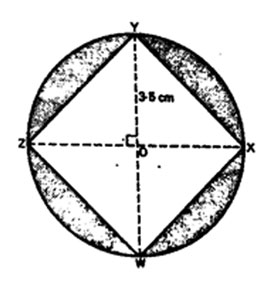What is the area of the shaded part? (Take 22/7)

A. 38.5 cm2      B. 26.25cm2     C. 24.5 cm2     D. 14.0 cm2

4. What is the area of the shaded region in the diagram below? (Take  22/7)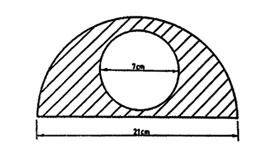A. 269.5 cm2     B. 211.75 cm2     C. 173.25 cm2     D. 134.75 cm2

5. The diagram below shows Kamau’s plot of land. The dimensions are as shown in the diagram.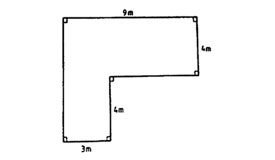What is the area of the plot?

A. 60 m2         B. 34 m2      C. 72 m2        D. 48 m2

6. What is the area of the shaded part in the figure below?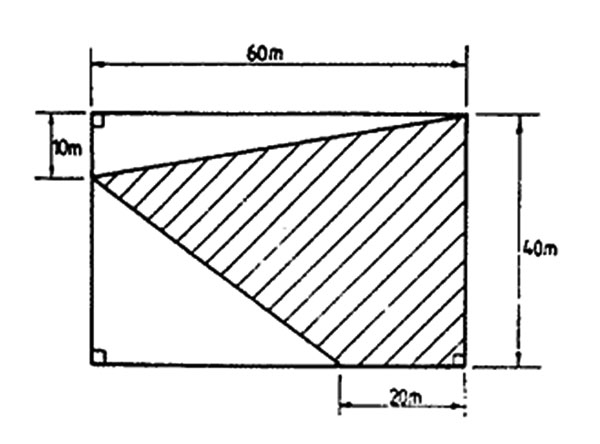A. 1200 m2        B. 1500 m2        C. 2400 m2       D. 900 m2

7. What is the area of the figure given below?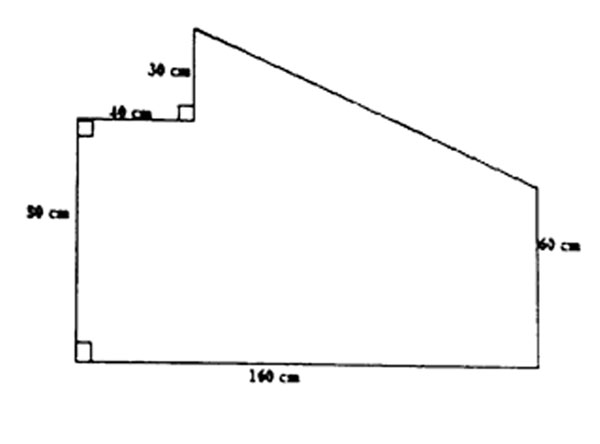A. 13400 cm2     B. 16400 cm2      C. 12200 cm2    D. 23600 cm2

8. The figure below represents a plot bound by two straight edges and a semi-circle.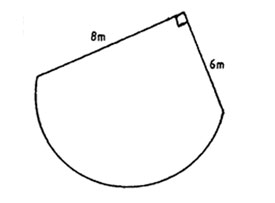What is the area of the plot? (Take 22/7)

A. 102.550 m2     B. 63.275 m2     C. 39.710 m2     D. 39.275 m2

9. The figure below represents a circle inscribed in a square of side 14 cm.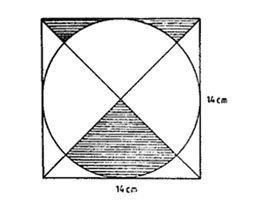What is the area of the shaded part in cm2? (Take 22/7)

A. 10.5       B. 38.5       C. 42.0       D. 49.0

10. The figure below represents a square enclosed in a circle of radius 7 cm.What is the area of the shaded parts? (Take 22/7)

A. 28 cm2      B. 56 cm2     C. 98 cm2       D. 154 cm2

11. In the figure below, XY, YZ, and XZ are diameters of circles.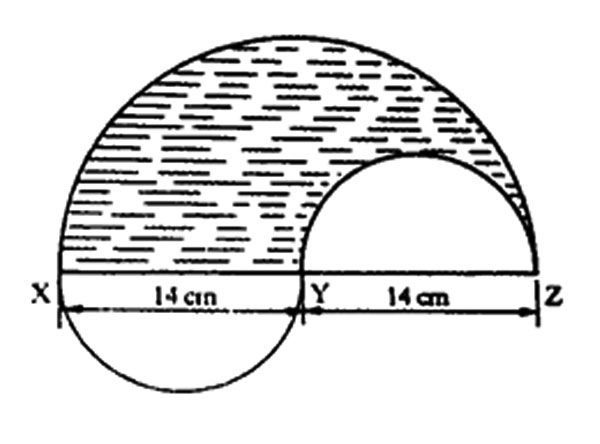What is the area of the shaded part? (Take 22/7)

A. 462 cm2      B. 385 cm2        C. 308 cm2      D. 231 cm2

12. The shaded part in the figure below represents Kamau’s plot of land.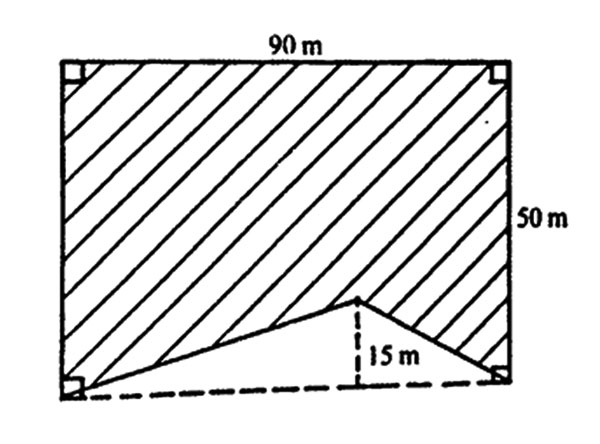What is the area of the plot in hectares?

A. 0.0675   B. 0.3150     C. 0.4500       D. 0.3825

13. In the diagram below, AB is the diameter of a semi-circle. AB = 10 cm, AC = 8 cm, BC = 6 cm and angle ACB = 900.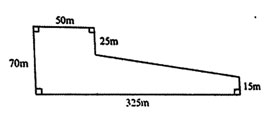What is the area of the shaded part? (Take 22/7)

A. 54.50 cm2      B. 39.25 cm2      C. 24.00 cm2      D. 15.25 cm2

14. The diagram below represents Mwau’s shamba. What is the size of Mwau’s shamba in hectares?

A. 1.175 ha      B. 1.325 ha       C. 2.0 ha       D. 11.75 ha

### Sources

• 71cd3aae-e62c-40f6-bd84-3179688e87de by elimu used under CC_BY-SA
• 23c82f06-c892-4009-9bd5-8b22832fd8e7 by elimu used under CC_BY-SA
• c980a754-b014-4504-9a44-deda962582e0 by elimu used under CC_BY-SA
• e56ecb18-9747-40a9-96d9-4eb8e9f6f838 by elimu used under CC_BY-SA
• 3af72df1-6c80-41f5-a6de-6b951fa4fc5e by elimu used under CC_BY-SA
• 848862c7-fbb5-41b4-80e1-6ad61ed6bd84 by elimu used under CC_BY-SA
• d850c7d5-210f-4bc6-b6db-126c7be00f42 by elimu used under CC_BY-SA
• 641b3946-c602-4ad3-95ff-37aaa8eac7cb by elimu used under CC_BY-SA
• fa8ba517-d583-4add-b159-f50c07582176 by elimu used under CC_BY-SA
• 7b732c98-24aa-4fcc-b365-a1229e82b278 by elimu used under CC_BY-SA
• fec1fd90-a693-424e-955c-e566c62e4848 by elimu used under CC_BY-SA
• f8752526-1dbd-41a7-80d9-b49aab5aa1f1 by elimu used under CC_BY-SA
• circle_area_and_cicumference by Unknown used under Creative Commons Attribution-NonCommercial-NoDerivatives 4.0 International License
• e9986892-b14f-4b77-9a51-bc25796cdc71 by elimu used under CC_BY-SA
• 540area by Unknown used under Creative Commons Attribution-NonCommercial-NoDerivatives 4.0 International License
• 1056_area by Unknown used under Creative Commons Attribution-NonCommercial-NoDerivatives 4.0 International License
• 1057_area by Unknown used under Creative Commons Attribution-NonCommercial-NoDerivatives 4.0 International License
• 1058_area by Unknown used under Creative Commons Attribution-NonCommercial-NoDerivatives 4.0 International License
• 1060area by Unknown used under Creative Commons Attribution-NonCommercial-NoDerivatives 4.0 International License
• 1061_area by Unknown used under Creative Commons Attribution-NonCommercial-NoDerivatives 4.0 International License
• a by Unknown used under Creative Commons Attribution-NonCommercial-NoDerivatives 4.0 International License
• b by Unknown used under Creative Commons Attribution-NonCommercial-NoDerivatives 4.0 International License
• 937fa9fe-5b4c-4c38-8e77-61451fb1570c by elimu used under CC_BY-SA
• circle_rectangle by Unknown used under Creative Commons Attribution-NonCommercial-NoDerivatives 4.0 International License
• area_combined_1 by Unknown used under Creative Commons Attribution-NonCommercial-NoDerivatives 4.0 International License
• window by Unknown used under Creative Commons Attribution-NonCommercial-NoDerivatives 4.0 International License
• window_solution by Unknown used under Creative Commons Attribution-NonCommercial-NoDerivatives 4.0 International License
• 08d76238-9fc6-498f-a3c7-33c01f8d4be1 by e-Limu used under CC_BY-SA
• 3f964aeb-69db-4f4e-b48c-8b5627e7dcd8 by e-Limu used under CC_BY-SA
• 5c4da086-1d9e-4ff7-a9b4-6b28be6b4e6d by e-Limu used under CC_BY-SA
• d3985ebc-8cd3-4f41-bd2a-51abdb557344 by e-Limu used under CC_BY-SA
• d8281ade-f797-4ea1-ae2d-652a709a9cfe by e-Limu used under CC_BY-SA
• 23d83671-6c97-4a57-b701-0befda71c90a by e-Limu used under CC_BY-SA
• 73bb2239-28c7-4e10-9535-db202973a43b by e-Limu used under CC_BY-SA
• 7c827cbc-8376-4909-8913-32482b60f9d7 by e-Limu used under CC_BY-SA
• 80e9613c-94ae-4158-b406-a659ee3c538f by e-Limu used under CC_BY-SA
• c9cab198-bf42-47a2-9b4c-3628dc91d0f4 by e-Limu used under CC_BY-SA
• e231e080-b908-4468-ba6e-1056f484b66f by e-Limu used under CC_BY-SA

•